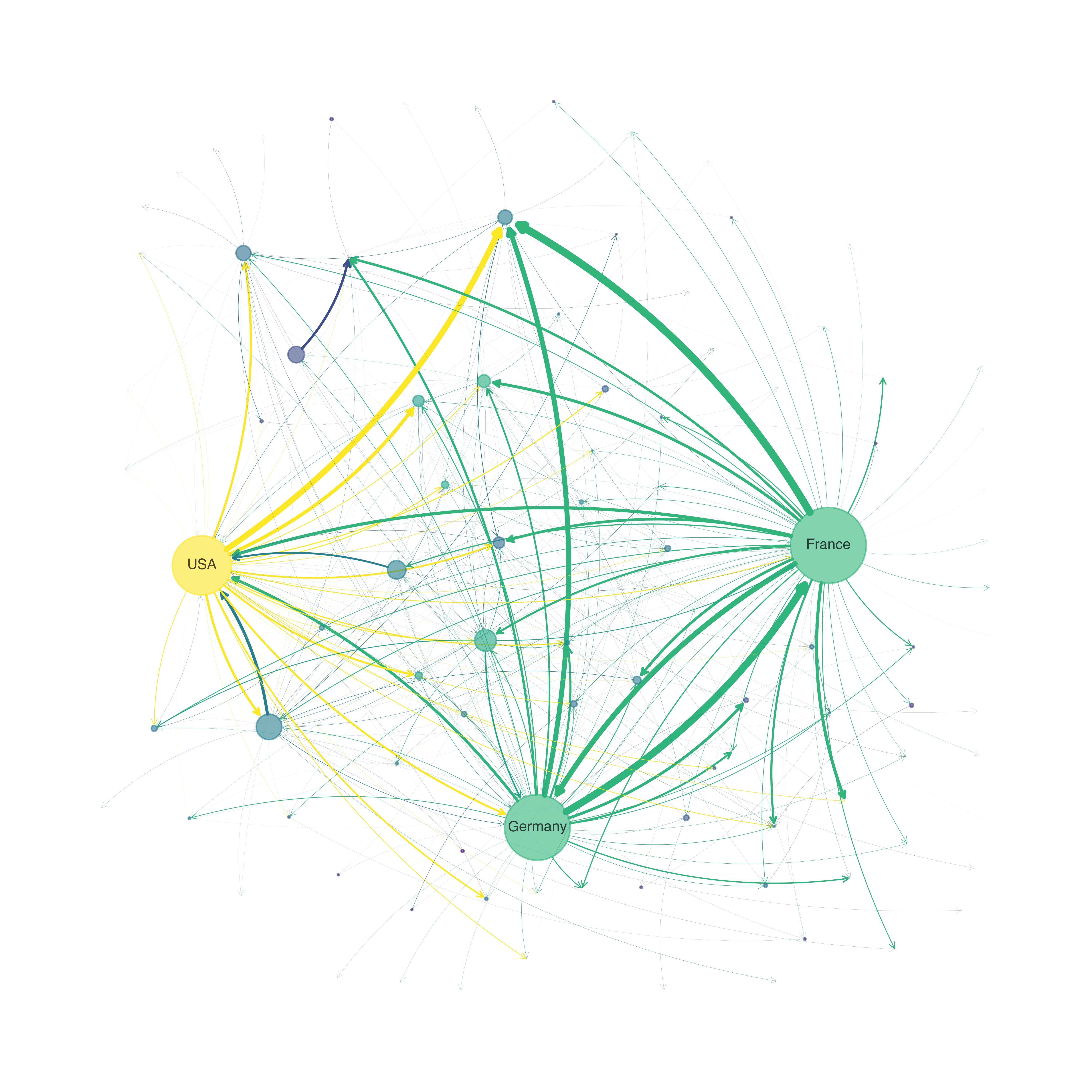# Economic Networks

## Theory and Computation## Introduction

This textbook is an introduction to economic networks, intended for students and researchers in the fields of economics and applied mathematics. The textbook emphasizes quantitative modeling, with the main underlying tools being graph theory, linear algebra, fixed point theory and programming. The text is suitable for a one-semester course, taught either to advanced undergraduate students who are comfortable with linear algebra or to beginning graduate students.

#### Chapter 1: Introduction

1. Motivation
2. Spectral Theory
3. Probability
4. Graph Theory

#### Chapter 2: Production

1. Multisector Models
2. General Equilibrium
3. More Spectral Theory

#### Chapter 3: Optimal Flows

1. Shortest Paths
2. Linear Programming and Duality
3. Optimal Transport

#### Chapter 4: Markov Chains and Networks

1. Markov Chains as Digraphs
2. Asymptotics

#### Chapter 5: Nonlinear Interactions

1. Fixed Point Theory
2. Financial Networks